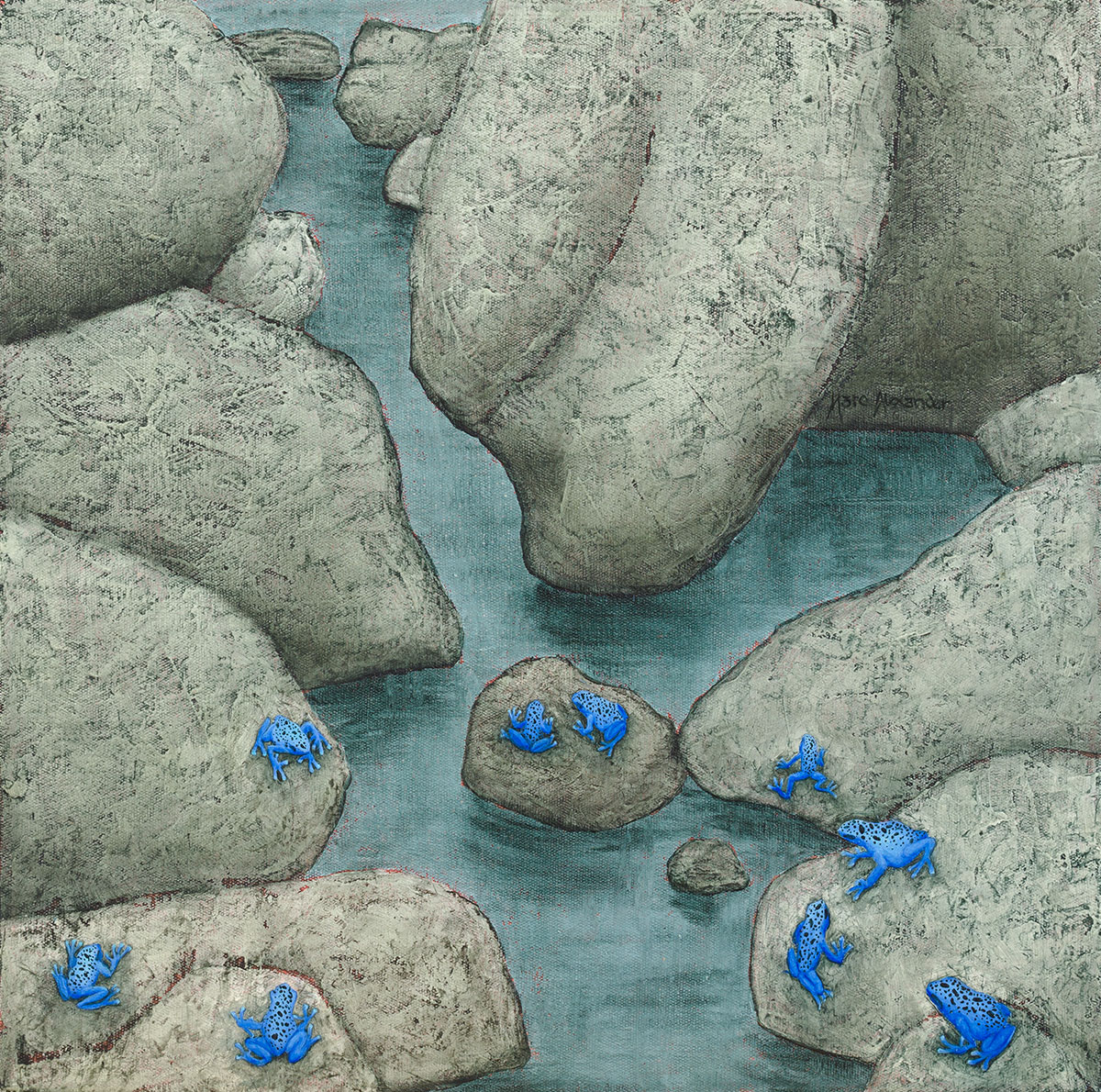##### Poison Arrow Frogs
Currency conversion from USD to: [convert number=505.00 from="usd" to="zar"] ZAR | [convert number=505.00 from="usd" to="gbp"] GBP | [convert number=505.00 from="usd" to="eur"] EUR     Medium: Oil on Canvas Size: 40cm by 40cm SOLD
\$ 505.00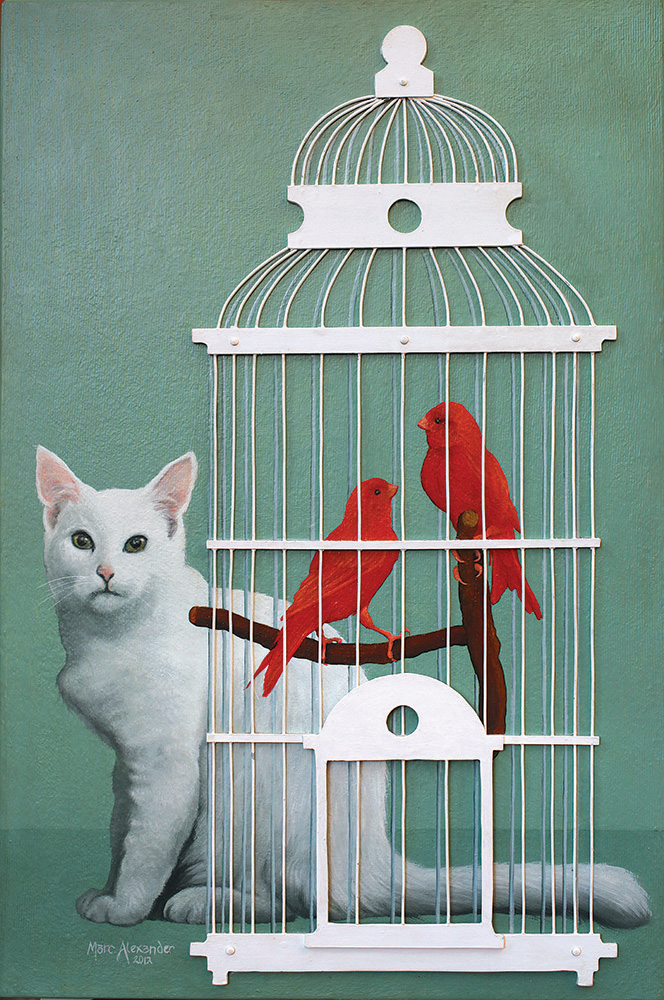##### Bird Cage I
Currency conversion from USD to: [convert number=1450.00 from="usd" to="zar"] ZAR | [convert number=1450.00 from="usd" to="gbp"] GBP | [convert number=1450.00 from="usd" to="eur"] EUR     Medium: Oil and wire on Canvas Size: 60cm by 40cm SOLD
\$ 1,050.00##### Crow’s Nest
Currency conversion from USD to: [convert number=1400.00 from="usd" to="zar"] ZAR | [convert number=1400.00 from="usd" to="gbp"] GBP | [convert number=1400.00 from="usd" to="eur"] EUR     Medium: Oil on Canvas Size: 40cm by 40cm SOLD
\$ 1,400.00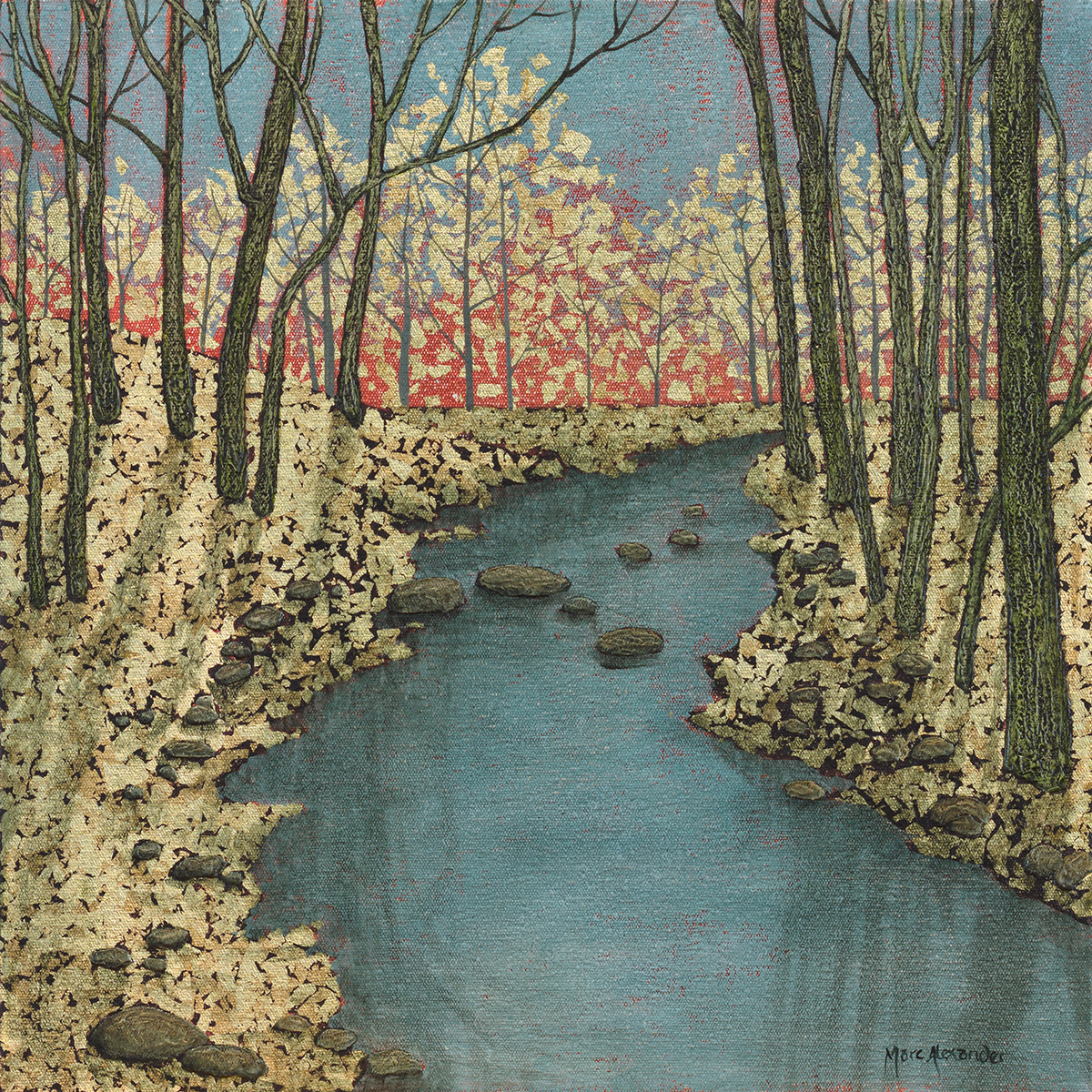##### Forest Stream I
Currency conversion from USD to: [convert number=1400.00 from="usd" to="zar"] ZAR | [convert number=1400.00 from="usd" to="gbp"] GBP | [convert number=1400.00 from="usd" to="eur"] EUR     Medium: Oil and Gold Leaf on Canvas Size: 40cm by 40cm SOLD
\$ 1,400.00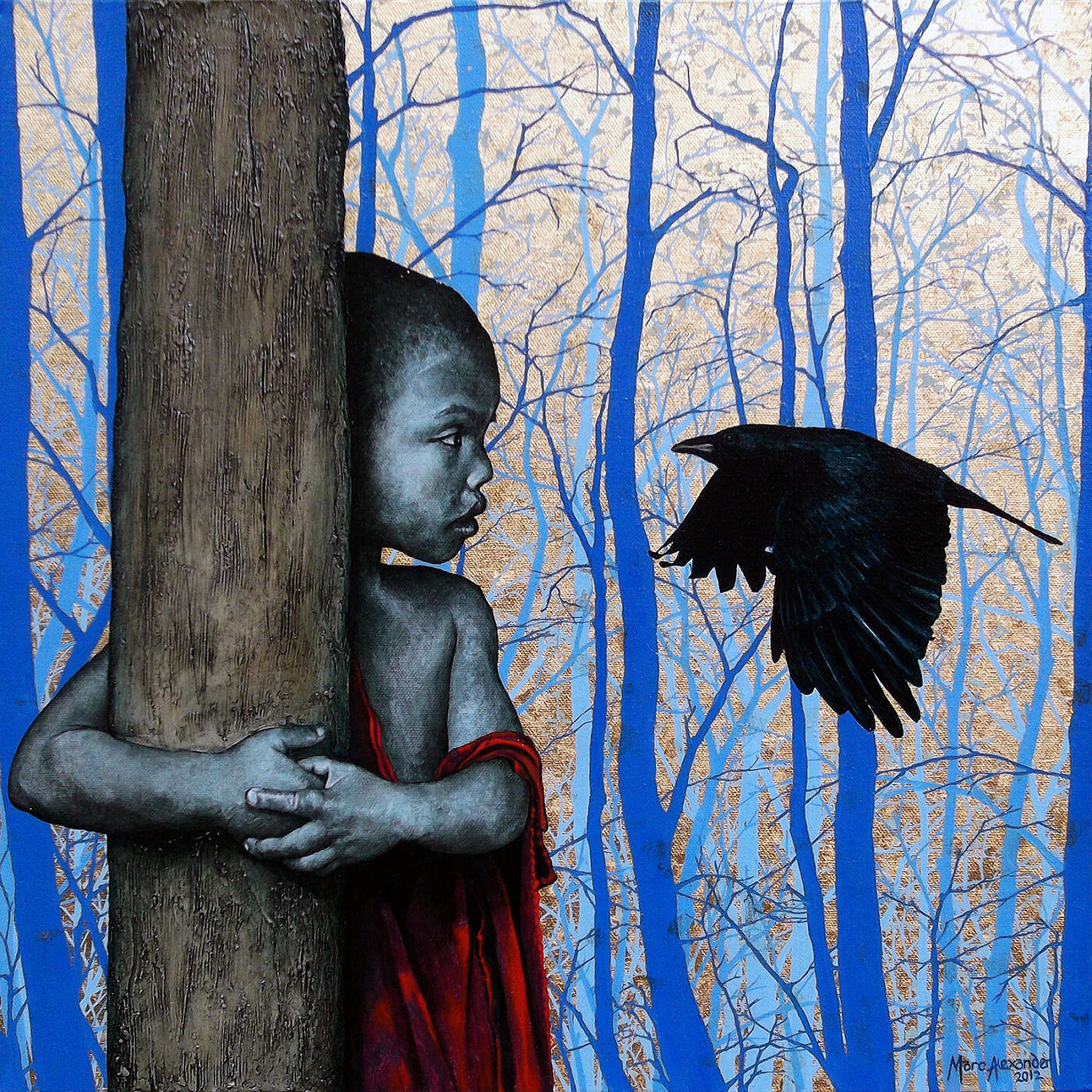##### Enchanted Forest I
Currency conversion from USD to: [convert number=1450.00 from="usd" to="zar"] ZAR | [convert number=1450.00 from="usd" to="gbp"] GBP | [convert number=1450.00 from="usd" to="eur"] EUR     Medium: Oil Gold Leaf on Canvas Size: 50cm by 50cm SOLD
\$ 1,450.00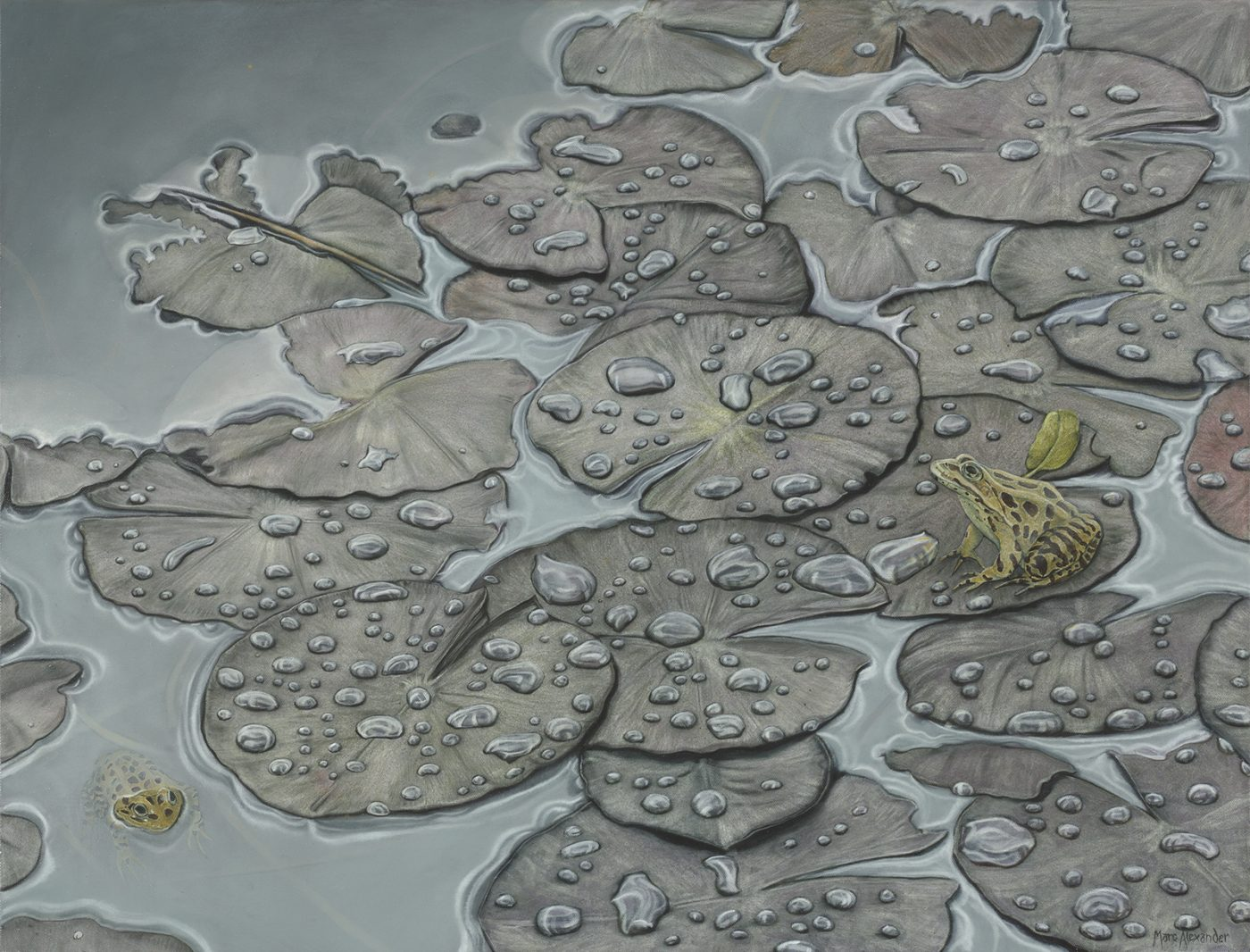Currency conversion from USD to: [convert number=1490.00 from="usd" to="zar"] ZAR | [convert number=1490.00 from="usd" to="gbp"] GBP | [convert number=1490.00 from="usd" to="eur"] EUR   Medium: Oil on Canvas Size: 68cm by 88cm SOLD
\$ 1,490.00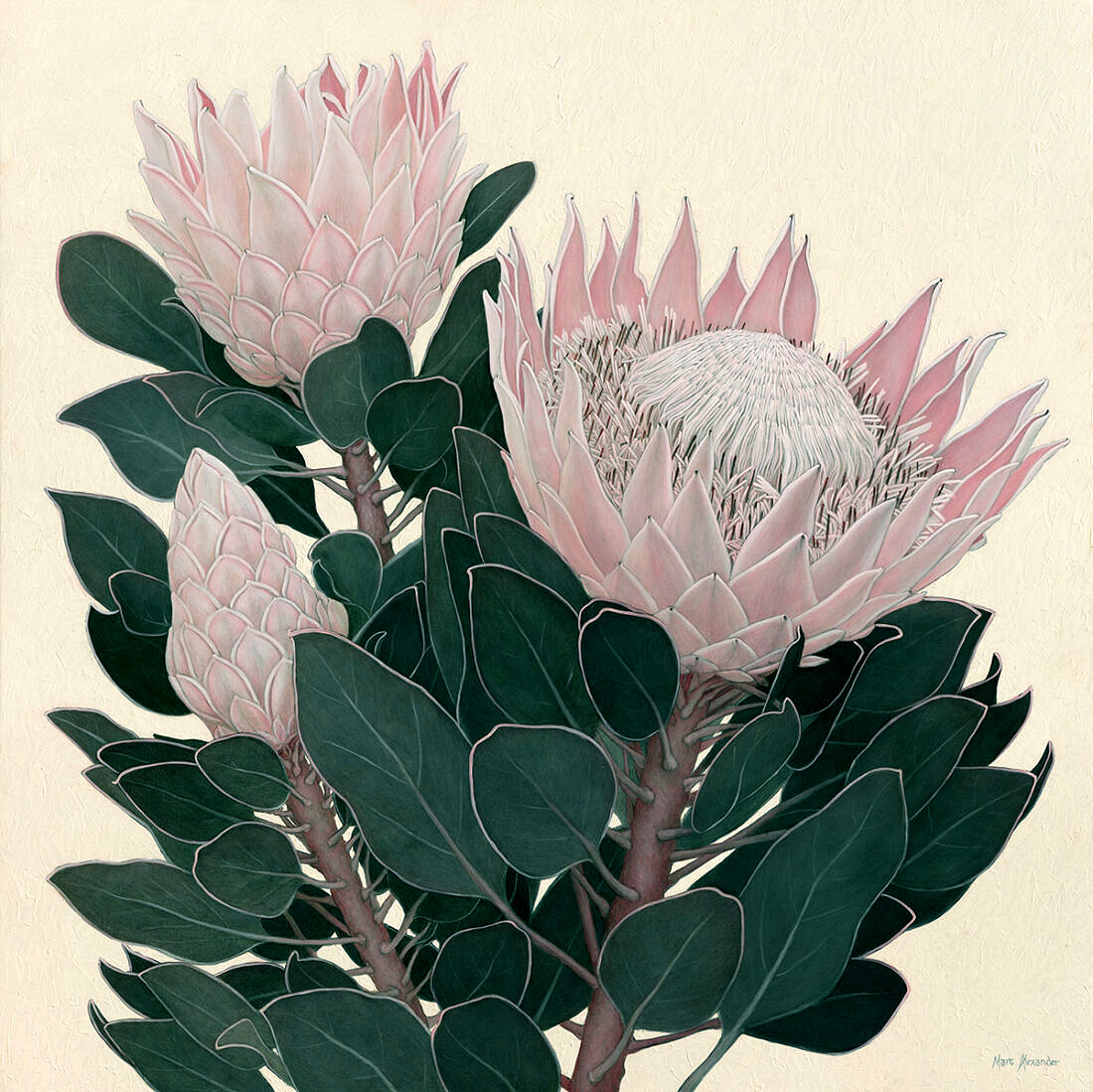##### King Protea I
Currency conversion from USD to: [convert number=2300.00 from="usd" to="zar"] ZAR | [convert number=2300.00 from="usd" to="gbp"] GBP | [convert number=2300.00 from="usd" to="eur"] EUR     Medium: Oil on Canvas Size: 100cm by 100cm SOLD
\$ 2,300.00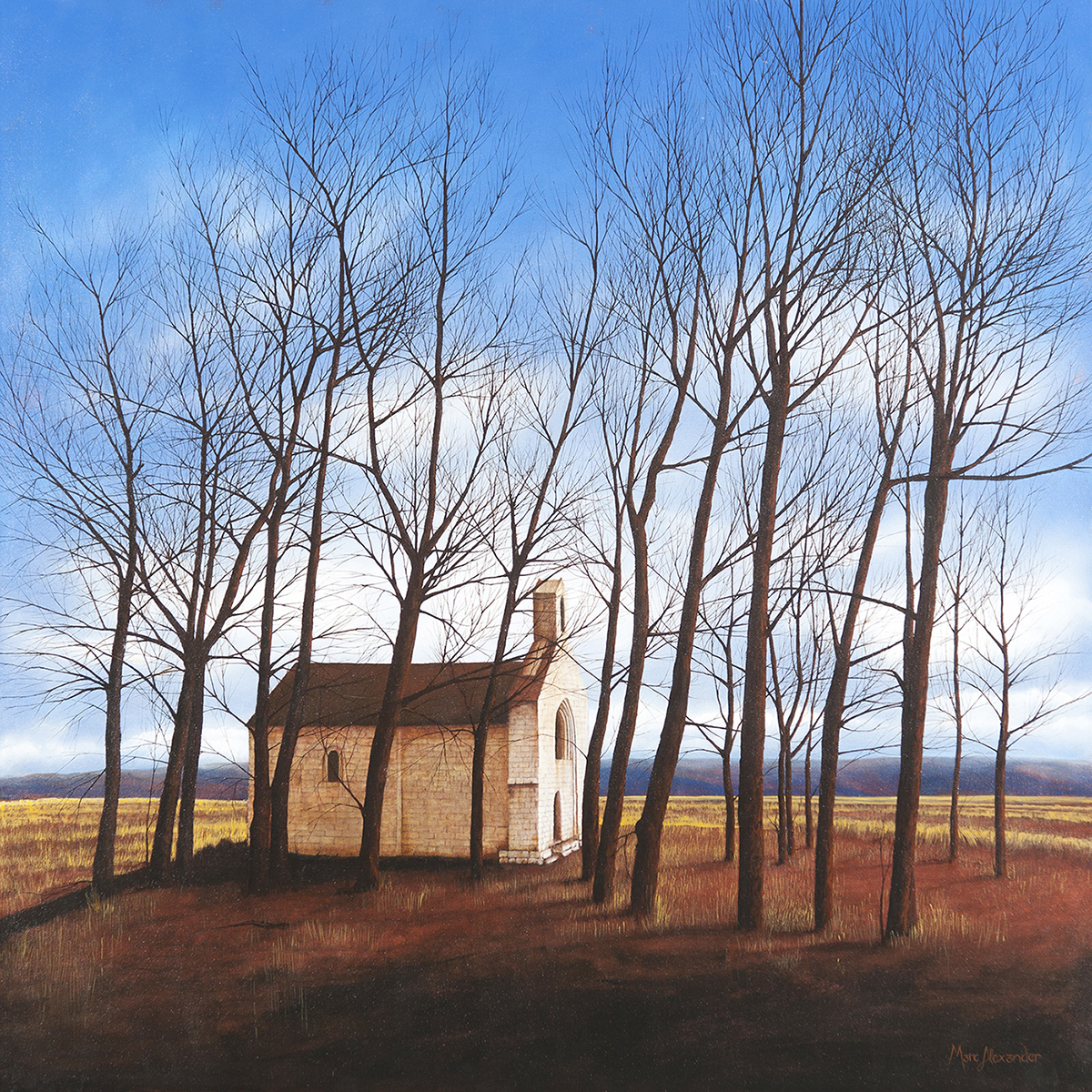##### Winter
Currency conversion from USD to: [convert number=2500.00 from="usd" to="zar"] ZAR | [convert number=2500.00 from="usd" to="gbp"] GBP | [convert number=2500.00 from="usd" to="eur"] EUR     Medium: Oil on Canvas Size: 100cm by 100cm SOLD
\$ 2,500.00##### Spring
Currency conversion from USD to: [convert number=2500.00 from="usd" to="zar"] ZAR | [convert number=2500.00 from="usd" to="gbp"] GBP | [convert number=2500.00 from="usd" to="eur"] EUR     Medium: Oil on Canvas Size: 100cm by 100cm SOLD
\$ 2,500.00##### Snow Leopard II
Currency conversion from USD to: [convert number=10050.00 from="usd" to="zar"] ZAR | [convert number=10050.00 from="usd" to="gbp"] GBP | [convert number=10050.00 from="usd" to="eur"] EUR     Medium: Oil on Canvas Size: 120cm by 200cm SOLD
\$ 10,050.00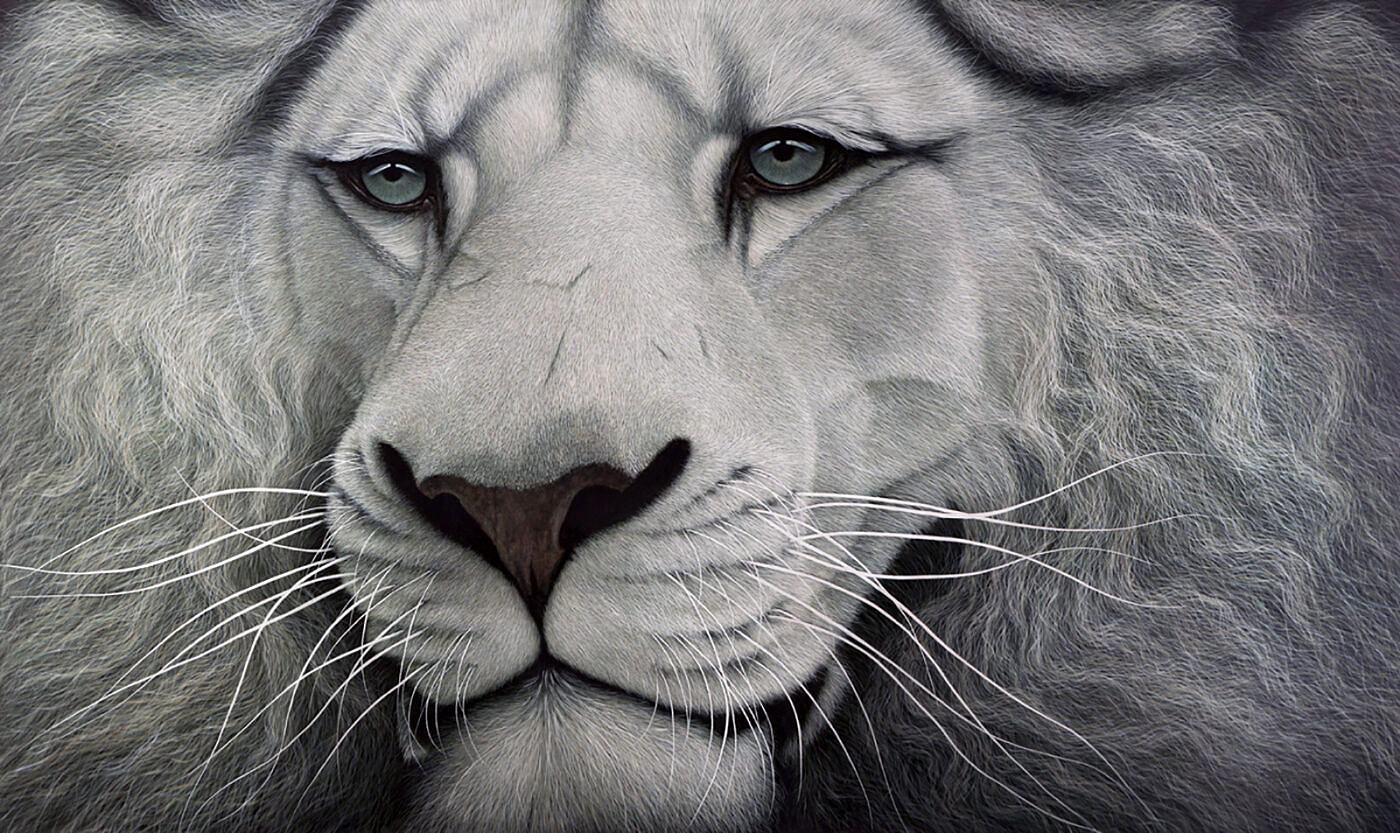##### White Lion
Currency conversion from USD to: [convert number=11000.00 from="usd" to="zar"] ZAR | [convert number=11000.00 from="usd" to="gbp"] GBP | [convert number=11000.00 from="usd" to="eur"] EUR     Medium: Oil on Canvas Size: 120cm by 200cm SOLD
\$ 11,000.00